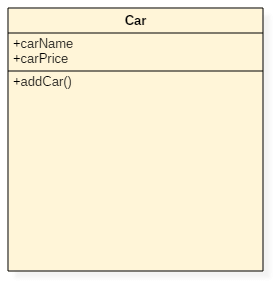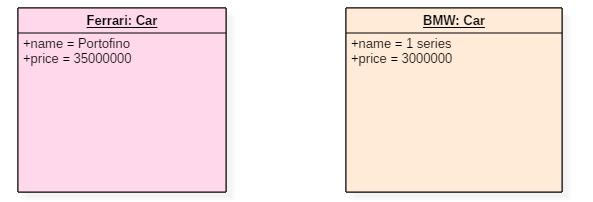Before we learn Object Diagram, let's understand-

## What is a Class Diagram?

A class represents things that are put together having common behavior. It is a blueprint of an object-oriented system

Let us consider an exciting example of classes and objects. We all know cars like Ferrari, Volkswagen, Mercedes, and Porsche, etc. These are all cars manufacturing companies. Here, we can consider that a car is a class and Ferrari, Mercedes are the objectsClass Diagram Notation

In this tutorial, you will learn:

## What is an Object Diagram?

Objects are the real-world entities whose behavior is defined by the classes. Objects are used to represent the static view of an object-oriented system. We cannot define an object without its class. Object and class diagrams are somewhat similar.

The difference between the class and object diagram is that the class diagram mainly represents the bird's eye view of a system which is also referred to as an abstract view. An object diagram describes the instance of a class. It visualizes the particular functionality of a system.

### Notation of an object diagram:Object Notation

## How to draw an object diagram?

1. Before drawing an object diagram, one should analyze all the objects inside the system.
2. The relations of the object must be known before creating the diagram.
3. Association between various objects must be cleared before.
4. An object should have a meaningful name that describes its functionality.
5. An object must be explored to analyze various functionalities of it.

## Purpose of an object diagram:

1. It is used to describe the static aspect of a system.
2. It is used to represent an instance of a class.
3. It can be used to perform forward and reverse engineering on systems.
4. It is used to understand the behavior of an object.
5. It can be used to explore the relations of an object and can be used to analyze other connecting objects.

### Object diagram example:Object Diagram

The above UML object diagram contains two objects named Ferrari and BMW which belong to a class named as a Car. The objects are nothing but real-world entities that are the instances of a class.

## Applications of Object Diagrams:

1. Object diagrams play an essential role while generating a blueprint of an object-oriented system.
2. Object diagrams provide means of modeling the classes, data and other information as a set or a single unit.
3. It is used for analyzing the online or offline system. The functioning of a system can be visualized using object diagrams.

## Class vs. Object Diagrams

 Serial no. Class Object 1 It represents static aspects of a system. It represents the behavior of a system in real time. 2 It doesn't include dynamic changes. It captures runtime changes of a system. 3 It never includes attributes or data values of an instance. It includes attributes and data values of any instance. 4 Class diagram manipulates the behavior of objects. Objects are instances of classes.

## Summary

1. Class groups together things that share similar behavior.
2. A class represents a bird's eye view of a system, i.e., an abstraction which is an object-oriented programming concept.
3. An object represents a static view of an object-oriented system.
4. One class can refer to multiple classes.
5. A single class can have any number of objects.
6. Objects are related to one another because they share the same class.
7. The object of different classes can also be connected.

YOU MIGHT LIKE: